# 跨平台智能交易系统: 时间过滤器

28 八月 2017, 07:48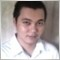0
4 810

### 概述

1. 规避某些时间段 (如, 横盘走势或高波动的时间段)
2. 为市场订单或持仓设置 "到期" 时间 (在 "到期" 时离场)
3. 交易周结束时平仓。

### 目标

• 理解并应用最常用的时间过滤方法
• 允许智能交易系统轻松使用多个时间过滤器
• 与 MQL4 和 MQL5 兼容

### 基类

```class CTimeBase : public CObject
{
protected:
bool              m_active;
bool              m_reverse;
CSymbolManager   *m_symbol_man;
CEventAggregator *m_event_man;
CObject          *m_container;
public:
CTimeBase(void);
~CTimeBase(void);
virtual int       Type(void) const {return CLASS_TYPE_TIME;}
//--- 初始化
virtual bool      Init(CSymbolManager*,CEventAggregator*);
virtual CObject *GetContainer(void);
virtual void      SetContainer(CObject*);
virtual bool      Validate(void);
//--- 赋值与取值
bool              Active(void) const;
void              Active(const bool);
bool              Reverse(void);
void              Reverse(const bool);
//--- 检查
virtual bool      Evaluate(datetime)=0;
};
```

### 时间过滤器类和类型

#### 按特定日期范围进行时间过滤

```class CTimeRangeBase : public CTime
{
protected:
datetime          m_begin;
datetime          m_end;
public:
CTimeRangeBase(void);
CTimeRangeBase(datetime,datetime);
~CTimeRangeBase(void);
//--- 初始化                    datetime,datetime
virtual bool      Set(datetime,datetime);
virtual bool      Validate(void);
//--- 赋值与取值
datetime          Begin(void) const;
void              Begin(const datetime);
datetime          End(void) const;
void              End(const datetime);
//--- 处理
virtual bool      Evaluate(datetime);
};
```

```bool CTimeRangeBase::Evaluate(datetime current=0)
{
if(!Active())
return true;
if(current==0)
current=TimeCurrent();
bool result=current>=m_begin && current<m_end;
return Reverse()?!result:result;
}
```

#### 按周内所在日进行时间过滤

```class CTimeDaysBase : public CTime
{
protected:
long              m_day_flags;
public:
CTimeDaysBase(void);
CTimeDaysBase(const bool sun=false,const bool mon=true,const bool tue=true,const bool wed=true,
const bool thu=true,const bool fri=true,const bool sat=false);
~CTimeDaysBase(void);
//--- 初始化
virtual bool      Validate(void);
virtual bool      Evaluate(datetime);
virtual void      Set(const bool,const bool,const bool,const bool,const bool,const bool,const bool);
//--- 赋值与取值
bool              Sunday(void) const;
void              Sunday(const bool);
bool              Monday(void) const;
void              Monday(const bool);
bool              Tuesday(void) const;
void              Tuesday(const bool);
bool              Wednesday(void) const;
void              Wednesday(const bool);
bool              Thursday(void) const;
void              Thursday(const bool);
bool              Friday(void) const;
void              Friday(const bool);
bool              Saturday(void) const;
void              Saturday(const bool);
};
```

```enum ENUM_TIME_DAY_FLAGS
{
TIME_DAY_FLAG_SUN=1<<0,
TIME_DAY_FLAG_MON=1<<1,
TIME_DAY_FLAG_TUE=1<<2,
TIME_DAY_FLAG_WED=1<<3,
TIME_DAY_FLAG_THU=1<<4,
TIME_DAY_FLAG_FRI=1<<5,
TIME_DAY_FLAG_SAT=1<<6
};
```

```void CTimeDaysBase::Set(const bool sun=false,const bool mon=true,const bool tue=true,const bool wed=true,
const bool thu=true,const bool fri=true,const bool sat=false)
{
Sunday(sun);
Monday(mon);
Tuesday(tue);
Wednesday(wed);
Thursday(thu);
Friday(fri);
Saturday(sat);
}

```

```void CTimeDaysBase::Monday(const bool set)
{
if(set)
m_day_flags|=TIME_DAY_FLAG_MON;
else
m_day_flags &=~TIME_DAY_FLAG_MON;
}
```

```bool CTimeDaysBase::Evaluate(datetime current=0)
{
if(!Active())
return true;
bool result=false;
MqlDateTime time;
if(current==0)
current=TimeCurrent();
TimeToStruct(current,time);
switch(time.day_of_week)
{
case 0: result=Sunday();      break;
case 1: result=Monday();      break;
case 2: result=Tuesday();     break;
case 3: result=Wednesday();   break;
case 4: result=Thursday();    break;
case 5: result=Friday();      break;
case 6: result=Saturday();    break;
}
return Reverse()?!result:result;
}
```

#### 使用计时器

```class CTimerBase : public CTime
{
protected:
uint              m_years;
uint              m_months;
uint              m_days;
uint              m_hours;
uint              m_minutes;
uint              m_seconds;
int               m_total;
int               m_elapsed;
datetime          m_time_start;
public:
CTimerBase(const int);
CTimerBase(const uint,const uint,const uint,const uint,const uint,const uint);
~CTimerBase(void);
//--- 初始化
virtual bool      Set(const uint,const uint,const uint,const uint,const uint,const uint);
virtual bool      Validate(void);
//--- 赋值与取值
uint              Year(void) const;
void              Year(const uint);
uint              Month(void) const;
void              Month(const uint);
uint              Days(void) const;
void              Days(const uint);
uint              Hours(void) const;
void              Hours(const uint);
uint              Minutes(void) const;
void              Minutes(const uint);
uint              Seconds(void) const;
void              Seconds(const uint);
bool              Total(void) const;
datetime          TimeStart(void) const;
void              TimeStart(const datetime);
//--- 处理
virtual bool      Elapsed(void) const;
virtual bool      Evaluate(datetime);
virtual void      RecalculateTotal(void);
};
```

```#define YEAR_SECONDS 31536000
#define MONTH_SECONDS 2419200
#define DAY_SECONDS 86400
#define HOUR_SECONDS 3600
#define MINUTE_SECONDS 60
```

```CTimerBase::CTimerBase(const uint years,const uint months,const uint days,const uint hours,const uint minutes,const uint seconds) : m_years(0),
m_months(0),
m_days(0),
m_hours(0),
m_minutes(0),
m_seconds(0),
m_total(0),
m_time_start(0)
{
Set(years,months,days,hours,minutes,seconds);
}
```

```CTimerBase::CTimerBase(const int total_time) : m_years(0),
m_months(0),
m_days(0),
m_hours(0),
m_minutes(0),
m_seconds(0),
m_total(0),
m_time_start(0)
{
m_total=total_time;
}
```

```datetime CTimerBase::TimeStart(void) const
{
return m_time_start;
}

void CTimerBase::TimeStart(const datetime time_start)
{
m_time_start=time_start;
}
```

```bool CTimerBase::Evaluate(datetime current=0)
{
if(!Active())
return true;
bool result=true;
if(current==0)
current= TimeCurrent();
m_elapsed=(int)(current-m_time_start);
if(m_elapsed>=m_total) result=false;
return Reverse()?!result:result;
}
```

#### 使用日内时间表进行过滤

```class CTimeFilterBase : public CTime
{
protected:
MqlDateTime       m_filter_start;
MqlDateTime       m_filter_end;
CArrayObj         m_time_filters;
public:
CTimeFilterBase(void);
CTimeFilterBase(const int,const int,const int,const int,const int,const int,const int);
~CTimeFilterBase(void);
virtual bool      Init(CSymbolManager*,CEventAggregator*);
virtual bool      Validate(void);
virtual bool      Evaluate(datetime);
virtual bool      Set(const int,const int,const int,const int,const int,const int,const int);
virtual bool      AddFilter(CTimeFilterBase*);
};
```

```bool CTimeFilterBase::Set(const int gmt,const int starthour,const int endhour,const int startminute=0,const int endminute=0,
const int startseconds=0,const int endseconds=0)
{
m_filter_start.hour=starthour+gmt;
m_filter_start.min=startminute;
m_filter_start.sec=startseconds;
m_filter_end.hour=endhour+gmt;
m_filter_end.min=endminute;
m_filter_end.sec=endseconds;
return true;
}
```

1. 以小时、分和秒表达所示时间, 然后将其与结构参数进行比较。
2. 用当前时间更新缺省的结构参数, 将结构转换为 UNIX 时间 ( datetime 类型), 并将其与所示时间进行比较。

```bool CTimeFilterBase::Evaluate(datetime current=0)
{
if(!Active())
return true;
bool result=true;
MqlDateTime time;
if(current==0)
current=TimeCurrent();
TimeToStruct(current,time);
m_filter_start.year= time.year;
m_filter_start.mon = time.mon;
m_filter_start.day = time.day;
m_filter_start.day_of_week = time.day_of_week;
m_filter_start.day_of_year = time.day_of_year;
m_filter_end.year= time.year;
m_filter_end.mon = time.mon;
m_filter_end.day = time.day;
m_filter_end.day_of_week = time.day_of_week;
m_filter_end.day_of_year = time.day_of_year;
/*
其它任务在这里处理
*/
}
```

1. 开始时间是从当天 (或从指定时间开始的那天), 结束时间是第二天。
2. 开始时间是从昨天 (所示时间的前一天) 开始, 结束时间是当天 (或所示时间的那天)。

1. 如果开始时间在所示时间同日之下, 则结束时间加 1 天。
2. 如果结束时间在所示时间同日之下, 则开始时间减去 1 天。

```if(m_filter_start.hour>=m_filter_end.hour)
{
if(time.hour>=m_filter_start.hour)
{
m_filter_end.day++;
m_filter_end.day_of_week++;
m_filter_end.day_of_year++;
}
else if(time.hour<=m_filter_end.hour)
{
m_filter_start.day--;
m_filter_start.day_of_week--;
m_filter_start.day_of_year--;
}
}
```

### 时间过滤器容器

```class CTimesBase : public CArrayObj
{
protected:
bool              m_active;
int               m_selected;
CEventAggregator *m_event_man;
CObject          *m_container;
public:
CTimesBase(void);
~CTimesBase(void);
virtual int       Type(void) const {return CLASS_TYPE_TIMES;}
//-- 初始化
virtual bool      Init(CSymbolManager*,CEventAggregator*);
virtual CObject *GetContainer(void);
virtual void      SetContainer(CObject*);
virtual bool      Validate(void) const;
//--- 激活和蛰伏
bool              Active(void) const;
void              Active(const bool);
int               Selected(void);
//--- 检查
virtual bool      Evaluate(datetime) const;
//--- 复原
virtual bool      CreateElement(const int);
};
```

### 子过滤器 (CTimeFilter)

```CTimes times = new CTimes();
CTimeFilter time1 = new CTimeFilter(gmt,8,17);
times.Add(GetPointer(time1));
```

```CTimes times = new CTimes();
CTimeFilter time1 = new CTimeFilter(gmt,8,12);
CTimeFilter time2 = new CTimeFilter(gmt,13,17);
times.Add(GetPointer(time1));
times.Add(GetPointer(time2));
```

```if(!result)
{
for(int i=0;i<m_time_filters.Total();i++)
{
CTimeFilter *filter=m_time_filters.At(i);
if(filter.Evaluate(current))
{
return true;
}
}
}
```

```CTimes times = new CTimes();
CTimeFilter time1 = new CTimeFilter(gmt,8,12);
CTimeFilter time2 = new CTimeFilter(gmt,13,17);
CTimeFilter time0 = new CTimeFilter(gmt,0,0);
time0.Reverse();
time0.AddFilter(GetPointer(time1));
time0.AddFilter(GetPointer(time2));
times.Add(GetPointer(time0));
```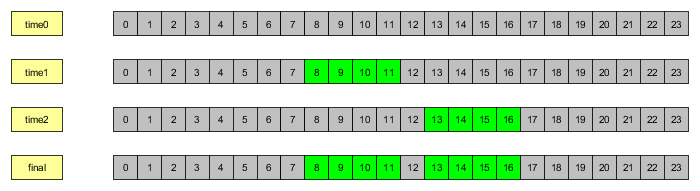### 示例

```#include "MQLx\Base\OrderManager\OrderManagerBase.mqh"
#include "MQLx\Base\Signal\SignalsBase.mqh"
#include "MQLx\Base\Time\TimesBase.mqh" //加入包含行
#include <Indicators\Custom.mqh>
```

`CTimes *time_filters;`

OnInit 下面, 我们创建一个 CTimes 的实例, 然后将其存储在这个指针上:

`time_filters = new CTimes();`

```if(signals.CheckOpenLong())
{
close_last();
if (time_filters.Evaluate(TimeCurrent()))
{
//Print("入场买入..");
money_manager.Selected(0);
order_manager.TradeOpen(Symbol(),ORDER_TYPE_BUY,symbol_info.Ask());
}
}
else if(signals.CheckOpenShort())
{
close_last();
if (time_filters.Evaluate(TimeCurrent()))
{
//Print("入场卖出..");
money_manager.Selected(1);
order_manager.TradeOpen(Symbol(),ORDER_TYPE_SELL,symbol_info.Bid());
}
}
```

```input bool time_range_enabled = true;
input datetime time_range_start = 0;
input datetime time_range_end = 0;
```

```if (time_range_enabled && time_range_end>0 && time_range_end>time_range_start)
{
CTimeRange *timerange = new CTimeRange(time_range_start,time_range_end);
time_filters.Add(GetPointer(timerange));
}
```

```input bool time_days_enabled = true;
input bool sunday_enabled = false;
input bool monday_enabled = true;
input bool tuesday_enabled = true;
input bool wednesday_enabled = true;
input bool thursday_enabled = true;
input bool friday_enabled = false;
input bool saturday_enabled = false;
```

```if (time_days_enabled)
{
CTimeDays *timedays = new CTimeDays(sunday_enabled,monday_enabled,tuesday_enabled,wednesday_enabled,thursday_enabled,friday_enabled,saturday_enabled);
time_filters.Add(GetPointer(timedays));
}
```

```input bool timer_enabled= true;
input int timer_minutes = 10080;
```

```if(timer_enabled)
{
CTimer *timer=new CTimer(timer_minutes*60);
timer.TimeStart(TimeCurrent());
time_filters.Add(GetPointer(timer));
}
```

1. 开始和结束时间在同一天内的时间过滤
2. 开始和结束时间不在同一天内的时间过滤
3. CTimeFilter 的多个实例

```enum ENUM_INTRADAY_SET
{
INTRADAY_SET_NONE=0,
INTRADAY_SET_1,
INTRADAY_SET_2
};
```

```input ENUM_INTRADAY_SET time_intraday_set=INTRADAY_SET_1;
input int time_intraday_gmt=0;
// 第一个集合
input int intraday1_hour_start=8;
input int intraday1_minute_start=0;
input int intraday1_hour_end=17;
input int intraday1_minute_end=0;
// 第二个集合
input int intraday2_hour1_start=8;
input int intraday2_minute1_start=0;
input int intraday2_hour1_end=12;
input int intraday2_minute1_end=0;
// 第三个集合
input int intraday2_hour2_start=13;
input int intraday2_minute2_start=0;
input int intraday2_hour2_end=17;
input int intraday2_minute2_end=0;
```

```switch(time_intraday_set)
{
case INTRADAY_SET_1:
{
CTimeFilter *timefilter=new CTimeFilter(time_intraday_gmt,intraday1_hour_start,intraday1_hour_end,intraday1_minute_start,intraday1_minute_end);
time_filters.Add(timefilter);
break;
}
case INTRADAY_SET_2:
{
CTimeFilter *timefilter=new CTimeFilter(0,0,0);
timefilter.Reverse(true);
CTimeFilter *sub1 = new CTimeFilter(time_intraday_gmt,intraday2_hour1_start,intraday2_hour1_end,intraday2_minute1_start,intraday2_minute1_end);
CTimeFilter *sub2 = new CTimeFilter(time_intraday_gmt,intraday2_hour2_start,intraday2_hour2_end,intraday2_minute2_start,intraday2_minute2_end);
timefilter.AddFilter(sub1);
timefilter.AddFilter(sub2);
time_filters.Add(timefilter);
break;
}
default: break;
}
```

```time_filters.Init(GetPointer(symbol_manager));
if(!time_filters.Validate())
{
Print("一个或多个时间过滤器验证失败");
return INIT_FAILED;
}
```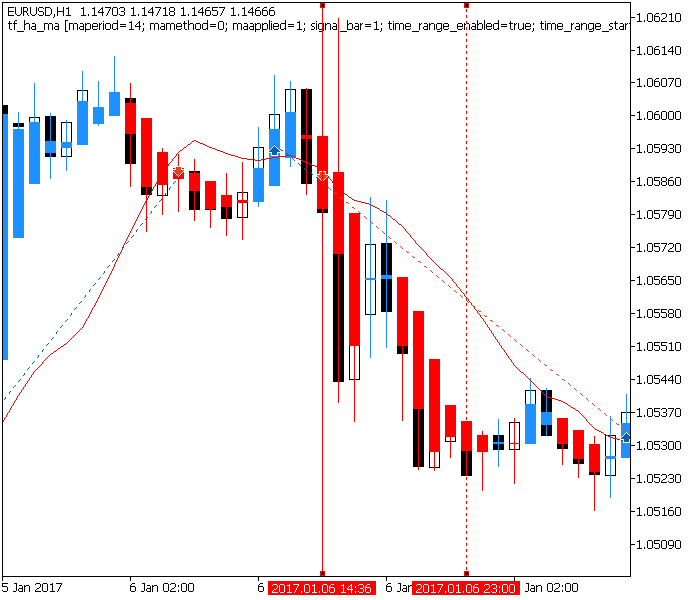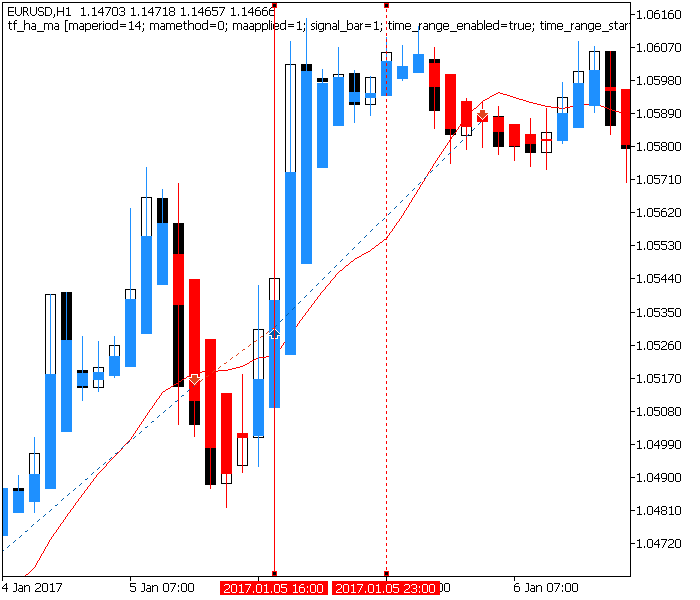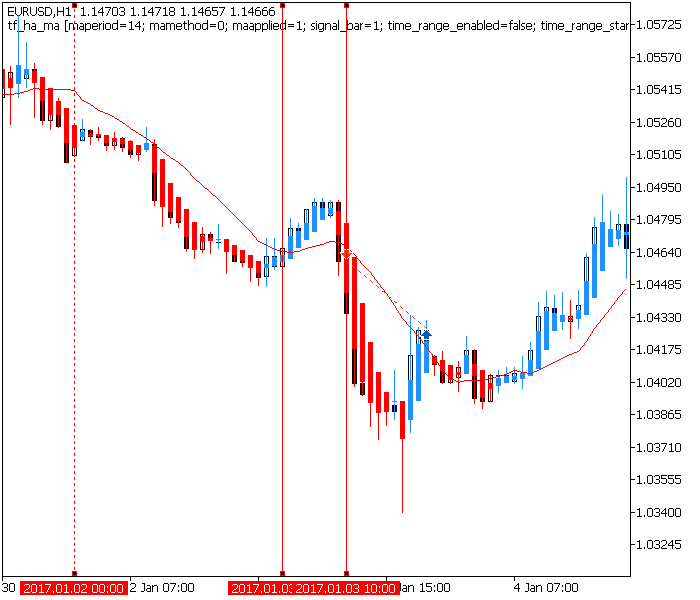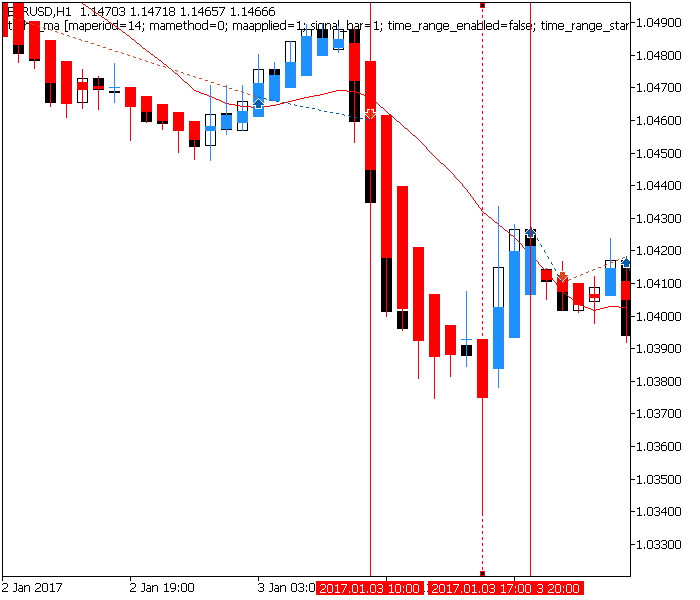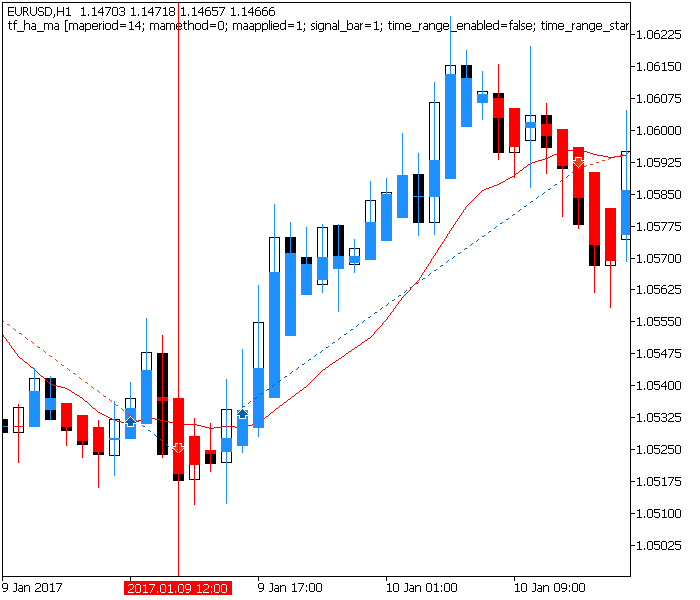### 结束语

tester_results.zip (464.13 KB)
tf_ha_ma.zip (1101.98 KB)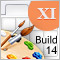图形界面 XI: 渲染控件 (统合构建14.2)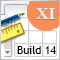图形界面 XI: 重构函数库代码 (集成编译 14.1)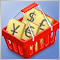交易货币对篮子时出现的测试形态。第 I 部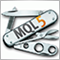通用EA交易: 访问交易品种的属性 (第8部分)